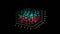Image by the author, generated with Python to illustrate a random number generation in the scatterplot.

# Random Number Generator Tutorial with Python

## Why are random numbers crucial in machine learning and data science? How do we build a random number generator for our projects?

Author(s): Sujan Shirol, Roberto Iriondo

This tutorial’s code is available on Github and its full implementation as well on Google Colab.

🤖 Towards AI is a community that discusses artificial intelligence, data science, data visualization, deep learning, machine learning, NLP, computer vision, related news, robotics, self-driving cars, programming, technology, and more! Join us🤖

Random numbers are everywhere in our lives, whether roulette in the Casino, cryptography, statistical sampling, or as simple as throwing a die gives us a random number between 1 to 6.

In this tutorial, we will dive into what pseudorandomness is, its importance in machine learning and data science, and how to create a random number generator to generate pseudorandom numbers in Python using popular libraries.

# What is Pseudorandomness?

To understand pseudorandomness first, we need to understand what randomness is. When we generate random numbers, they are a wholly unpredictable and non-deterministic sequence of numbers. There are two statistical properties of a sequence of random numbers:

1. Uniformity: The probability of occurrence of every number in an interval is the same.
2. Independence: The current random value has no relation with the previous random value.

As mentioned, the output of rolling a die or flipping a coin is “truly” random but at the same time mechanical, and hence, generating large samples of these needs a lot of time and work, which most of us do not possess.

Next, computers came along and made tasks easy and fast. However, computers cannot generate truly random numbers because computers are predictable, deterministic, and repeatable. They only do what we want them to do. That is why it is impossible to generate truly random numbers, and instead, computers give birth to pseudorandom numbers.

When we use a deterministic process to generate numbers, they appear close to randomness when we have a sufficiently large quantity of them.

To generate pseudorandom numbers, we need to initialize them with a seed. A seed is a truly random number, and it can be any whole number — for example, the current time in milliseconds.

There are several algorithms to generate pseudorandom numbers, and all of them initialize with a seed.

One of the earliest approaches was suggested by John von Neumann in the year 1946. He took an initial random number (seed), squared it, picked the middle digits of the resulting squared number, add it to a sequence of random numbers, squared the newly picked number, and the process continued.

# Importance of random numbers in data science and machine learning

Randomness is everywhere in the data science and machine learning domain, be it data collection, simulation, splitting data into train and test, data evaluation, algorithms, neural network, and others.

• How do we collect sample data? We pick truly random data points from the population, and the more random the points are, the more they represent the population. Several methods define how the random picking process must be: simple random sampling, systematic random sampling, stratified random sampling, and others.
• Sometimes we need not collect the actual data if we already know the distribution of the data we are supposed to deal with — saving a lot of time and effort. For example, suppose we know that the milage of a vehicle follows a normal distribution. We can generate random numbers of normal distribution for our study. This process is called simulation.
• We split the data into training and testing data. We use training data to train our model and consequently testing data to test the trained model. Generally, training data is 80%, and test data is 20% of the available data. This splitting of data has to be random for our model to perform efficiently.
• We evaluate our model for better performance by testing its accuracy on different random subsets of available data. Selecting these subsets is truly random-this process is called cross-validation.
• Randomness plays a critical role in most machine learning algorithms, like shuﬄing the training data before each epoch in stochastic gradient descent, random input features in random forest algorithm.
• The neural network algorithm starts with random initialization of weights and biases and then alters each epoch’s value to minimize error and increase accuracy.

# Pseudorandom number generators

A pseudorandom number generator (PRNG), also known as a deterministic random bit generator (DRBG), is an algorithm for generating a sequence of numbers whose properties approximate the properties of sequences of random numbers .

There are several PRNGs available. They are functions that we need to call, and it returns a random number. Every time we call the function, it returns a different random number depending on the seed value. Programming languages like Python allow us to get the randomness as an integer, floating-point, within a specific distribution, within a specific range, and so on . As we have already discussed, to generate pseudorandom numbers, the sequence has to be seeded with a number. The number can be any whole number. If the seed does not initialize explicitly, the default seed value is the current time in milliseconds.

Now that the theoretical concepts are clear, let‘s get into the coding part with Python examples to generate pseudorandom numbers.

# Generating pseudorandom numbers with Python’s standard library

Python has a built-in module called random to generate a variety of pseudorandom numbers. However, it is recommended that this module should not be used for security purposes, such as cryptography. However, using the standard library is perfect for machine learning and data science. This Python module uses a pseudorandom number generator (PRNG) called Mersenne Twister.

1. Initializing the generator: seed() is a function used to seed the generator. It takes an integer value. If we pass a string value, it is converted into an integer, and if no value is passed, the default value is the current system time.
`# importing the moduleimport random  # initialize the seed to 25random.seed(25)`

2. Random numbers within a range: randrange() and randint() are two functions that can be used interchangeably generating a random number within a specified range.

`random.seed(25)  # generate a random number between 10 and 20(both excluded)random.randrange(10, 20)  # generate a random number between 10 and 20(both included)random.randint(10, 20)`

Output:Figure 2: The output of our code snippet using the pseudorandom number generator (PRNG) called Mersenne Twister in Python.

3. Random element from a sequence: It is also possible to get a random element from a sequence, generally a list of any data type using the function choice().

`# initialize the seed to 2random.seed(2)  # setting up the sequencemyseq = ["Towards", "AI", "is", 1]  # randomly choosing an element from the sequencerandom.choice(myseq)`

Output:

4. Multiple random selections with different possibilities: Suppose we want multiple elements from the sequence chosen randomly, we use the function choices(). It also allows us to specify the weights, meaning, the possibility of occurrence of each element in the sequence. The random picking is down with replacement.

`# initialize the seed to 25random.seed(25)  # setting up the sequencemyseq = ["Towards", "AI", "is", 1]  # random selection of length 15# 10 time higher possibility of selecting 'Towards'# 5 time higher possibility of selecting 'AI'# 2 time higher possibility of selecting 'is'# 2 time higher possibility of selecting 1random.choices(myseq, weights=[10, 5, 2, 2], k=15)`

Output: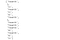Figure 4: The random output with multiple possibilities in a sequence.

5. Random element from a sequence without replacement: we have seen choice(), which selects elements randomly but with replacement, meaning the same element can be chosen multiple times. The function sample() is used to randomly select elements from a sequence without replacement, meaning, once an element is chosen it cannot appear again. So, the number of elements we choose to select should always be less than or equal to the original sequence's length.

`# initialize the seed to 25random.seed(25)  # setting up the sequencemyseq = ["Towards", "AI", "is", 1]  # randomly choosing an element from the sequencerandom.sample(myseq, 2)`

Output:

6. Rearranging the sequence: shuffle() is a function used to reorganize the sequence elements’ order.

`# initialize the seed to 25random.seed(25)  # setting up the sequencemyseq = ["Towards", "AI", "is", 1]  # rearranging the order of elements of the sequencerandom.shuffle(myseq)print(myseq)`

Output:

7. Floating-point random number: The function random() is used to get a random floating-point number between 0 and 1.

`#initialize the seed to 25random.seed(25)#random floating number between 0 and 1random.random()`

Output:

8. Real-value distributions: Many functions return real-value distributions. We will discuss uniform() and gauss(). uniform() returns a random floating-point number between specified ranges, including both upper and lower limits. gauss() returns a normal distribution floating-point number having specified mean and standard distribution.

`# initialize the seed to 25random.seed(25)  # random float number between 10 and 20 (both included)print(random.uniform(10, 20))  # random float number mean 10 standard deviation 4print(random.gauss(10, 4))`

Output:

# Generating pseudorandom numbers with NumPy

In machine learning, we are likely to use libraries such as scikit-learn and Keras. These libraries make use of NumPy under the covers, a library that makes working with vectors and matrices of numbers very efficient .

Numpy’s random number routines produce pseudorandom numbers using combinations of a BitGenerator to create sequences and a Generator to use those sequences to sample from different statistical distributions . As a programmer, we need not worry how it works under the hood.

Unlike the Python standard library, where we need to loop through the functions to generate multiple random numbers, NumPy always returns an array of both 1-D and 3-D random numbers without the need for looping. We will see how it works. It also allows us to generate random numbers from some popular statistical distributions like binomial, Poisson, chi-square, and others. A NumPy pseudorandom generator must initialize with a seed value; else, the current system time is used as a seed value.

1. Uniform distributed floating values: function rand() is used to generate uniformly distributed random floating-point numbers. It takes two arguments, row and column, specifying the numbers of rows and columns. If no argument is passed, it returns a single random number.
`# importing numpyimport numpy as np  # initialize the seed to 25np.random.seed(25)  # single uniformly distributed random numbernp.random.seed(25)np.random.rand()  # uniformly distributed random numbers of length 10: 1-D arraynp.random.seed(25)np.random.rand(    10)  # uniformly distributed random numbers of 2 rows and 3 columns: 2-D arraynp.random.seed(25)np.random.rand(2, 3)`

Output:

2. Normal distributed floating values: In these cases, we need normally distributed floating-point values. We use the function called randn(). This takes arguments as same as function rand() the only difference is the type of distribution.

`# initialize the seed to 25np.random.seed(25)  # single narmally distributed random numbernp.random.seed(25)np.random.randn()  # normally distributed random numbers of length 10: 1-D arraynp.random.seed(25)np.random.randn(    10)  # normally distributed random numbers of 2 rows and 3 columns: 2-D arraynp.random.seed(25)np.random.randn(2, 3)`

Output:

3. Uniform distributed integers in a given range: function randint() returns uniformly distributed integers for the specified range. Here, we use an argument called ‘size’ — which takes a tuple value specifying the dimensions of an array that is required.

`# initialize the seed to 25np.random.seed(25)  # single uniformly distributed random integer between 10 and 20np.random.randint(    10, 20)  # uniformly distributed random integer between 0 to 100 of length 10: 1-D arraynp.random.randint(    100, size=(10))  # uniformly distributed random integer between 0 to 100 of 2 rows and 3 columns: 2-D arraynp.random.randint(100, size=(2, 3))`

Output: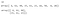Figure 11: Using NumPy with uniform distributed integers in a given range.

4. Random element from a defined list: NumPy also allows us to select one or more than one elements randomly from the defined list of elements of any datatype. It takes the defined list and size as an argument. If the size is not defined, it returns a single random element from the list by default.

`# initialize the seed to 25random.seed(25)  # setting up the sequencemyseq = ["Towards", "AI", "is", 1]  # randomly choosing an element from the sequencenp.random.choice(myseq)  # randomly choosing elements from the sequence: 2-D arrayrandom.seed(25)np.random.choice(myseq, size=(2, 3))`

Output: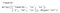Figure 12: Using NumPy to generate random numbers with a random element from a defined list.

We can also set the probability of occurrence of each element of the list while choosing it randomly. Remember, the given probability must sum to 1. For example, we set the probability for ‘Towards’ as 0.1, ‘AI’ as 0.6, ‘is’ as 0.05, and 1 as 0.25. Now 0.1+0.6+0.05+0.25 = 1. Since the occurrence probability of ‘AI’ is highest, we can see it appears the most number of times in the resultant array, followed by 1.

`# initialize the seed to 25random.seed(25)  # setting up the sequencemyseq = [    "Towards",    "AI",    "is",    1,]  # randomly choosing elements from the sequence with defined probabilities# The probability for the value to be 'Towards' is set to be 0.1# The probability for the value to be 'AI' is set to be 0.6# The probability for the value to be 'is' is set to be 0.05# The probability for the value to be 1 is set to be 0.25np.random.choice(myseq, p=[0.1, 0.6, 0.05, 0.25], size=(2, 3))`

Output: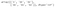Figure 13: Using defined probabilities to generate random numbers from a sequence with NumPy.

5. Binomial distributed values: the function binomial() takes three arguments n-number of trials, p-probability of occurrence of each trial, size-shape of the returned array. The returned array values are binomially distributed.

`# initialize the seed to 25np.random.seed(25)  # 10 number of trials with probability of 0.5 eachrandom.binomial(n=10, p=0.5, size=10)`

Output:

6. Poisson Distribution values: the function poisson() takes two arguments lam-rate, size-shape of the returned array. The returned array values are Poisson distributed. This estimated how many times an event can occur with a specified rate.

Output:

7. Chi-Square Distribution values: the function chisquare() is used to generate samples from the chi-square distribution. It takes two arguments df-degree of freedom and size-shape of the returned array.

`# initialize the seed to 25np.random.seed(25)  # degree of freedom 2 and size (2, 3)random.chisquare(df=2, size=(2, 3))`

Output:

# Summary

Thank you for reaching to this section of our random number generator tutorial. In this tutorial, we learned.

• What is randomness
• What is pseudorandomness
• Why is it impossible to generate truly random numbers
• Importance of randomness in machine learning and data science
• What is a pseudorandom number generator (PRNG)
• How to generate pseudorandom numbers using the Python standard library: random and NumPy

DISCLAIMER: The views expressed in this article are those of the author(s) and do not represent the views of Carnegie Mellon University nor other companies (directly or indirectly) associated with the author(s). These writings do not intend to be final products, yet rather a reflection of current thinking, along with being a catalyst for discussion and improvement.

All images are from the author(s) unless stated otherwise.

Published via Towards AI

# References

 Pseudorandom number generator, Wikipedia, https://en.wikipedia.org/wiki/Pseudorandom_number_generator

 Introduction to Random Number Generators for Machine Learning in Python, Machine Learning Mastery, https://machinelearningmastery.com/how-to-generate-random-numbers-in-python/

 Random sampling, NumPy Developer Docs, https://numpy.org/devdocs/reference/random/index.html

 How to generate random numbers in Python, Coding Ninjas, https://www.codingninjas.com/blog/2020/11/06/how-to-generate-random-number-in-python/

Written by

Written by

## Towards AI Team

#### Publishing the Best of Tech, Science, and Engineering | Editorial → https://towardsai.net/editorial | Subscribe→ https://towardsai.net/subscribe — @Towards_AI